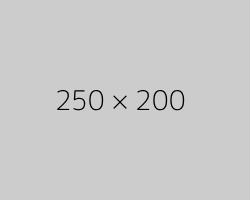# Eduvictors Class 10 Exercises and Solutions

Real Numbers: Important Formulas

## Real Numbers: Important Points and Formulae

1. Natural Numbers: These are counting numbers. N = {1,2,3,4,5...}

2. Whole numbers: Counting Numbers + Zero. W = {0,1,2,3,4,5...}

3. Integers: Negative and Positive Numbers. Z = {... -5, -4, -3, -2, -1, 0,1,2,3,4,5...}

4. Positive Integers: Z+ = {0,1,2,3,4,5...}

5. Negative Integers: Z- = {... -5, -4, -3, -2, -1}

6. Rational Numbers: A number is said to be rational number(Q) if it can be expressed in the form of $\frac{p}{q}$ where p and q are integers and q ≠ 0.
e.g. $\frac{1}{2},\frac{-3}{7}, 0, 7$ etc.

8. Irrational Numbers: A number is said to be irrational number if it cannot be expressed in the form of $\frac{p}{q}$
e.g. π, $\sqrt{2}...$

#### Euclid’s Division Lemma

❍   An algorithm is a series of well defined steps which gives a procedure for solving a type of problem.

❍   A lemma is a proven statement used for proving another statement.

❍   Euclid’s division algorithm is a technique to compute the Highest Common Factor $(HCF)$ of two given positive integers.

❍   To obtain the HCF of two positive integers, say c and d, with c > d, follow the steps below:

Step 1:
Apply Euclid’s division lemma, to c and d. So, we find whole numbers, q and r such that c = dq + r, 0 ≤ r < d.

Step 2:
If r = 0, d is the HCF of c and d. If r ≠ 0, apply the division lemma to d and r.

Step 3:
Continue the process till the remainder is zero. The divisor at this stage will be the required HCF.

#### Properties of HCF and LCM of two numbers 'a' and 'b'

$HCF(a, b)$ = Product of the smallest power of each common prime factor in the numbers.
$LCM(a, b)$ = Product of the greatest power of each prime factor, involved in the numbers.

If $HCF(a,b) = 1$, then a and b are co-primes.

$HCF( a , b ) \times LCM ( a , b ) = a \times b$

#### The Fundamental Theorem of Arithmetic

Every composite number can be expressed $(factorized)$ as a product of primes, and this factorization is unique, apart from the order in which the prime factors occur.

#### Irrationality of Square Roots of 2, 3 and 5

●   Let p be a prime number. If p divides a² , then p divides a, where a is a positive integer.

●   $\sqrt{2},\sqrt{3},\sqrt{5}$ are irrational

#### Decimal Expansions of Rational Numbers

●   Let x be a rational number whose decimal expansion terminates. Then we can express x in the form pq, where p and q are coprime, and the prime factorization of q is of the form 2n 5m, where n, m are non-negative integers

●   Let x = pq be a rational number, such that the prime factorization of q is of the form 2n 5m, where n, m are non-negative integers. Then x has a decimal expansion which terminates.

●   Let x = pq be a rational number, such that the prime factorization of q is not of the form 2n 5m, where n, m are non-negative integers. Then x has a decimal expansion which is non-terminating repeating $(recurring)$.